# Linear Hill Muscle### Watch the video tutorial on the muscle model!### Look here for other video tutorials using muscles!

Free C++ source code for the Hill muscle model is available.

There are two different types of models that are typically used to simulate the biomechanics of muscle. The first of these is the Hill muscle model (Hill 1938; Hill 1970). It is a purely mechanical muscle model built from the systems engineering perspective. The early pioneers in the field of muscle mechanics knew next to nothing about the internal structure and functioning of the muscle, so they had to treat it as a black box and attempted to map the input-output characteristics of muscle in an effort to formulate a mathematical model that could predict its overall behavior. The second type of muscle model was formulated once the sliding filament theory was proposed by Huxley (Huxley and Simmons 1971). It was built using a reductionist approach that takes into account the actual molecular structure of the muscle and attempts to predict the developed tension by simulating the forces produced by the crossbridge attachments between the actin and myosin molecules.

When building a biomechanical simulation of muscle a few key goals must be kept in mind. The primary goal is that the muscle model generates outputs that are a reasonable reproduction of what a real muscle would do in a similar situation. The biomechanical model does not have to produce the exact behavior, but it should be close within some well-defined range of error. Also, it should never produce results that are far outside the biologically realistic range of outputs for any situation, and it must be capable of functioning over the entire operating range of the real muscle. The model should also be as simple and fast as is feasible while maintaining biological realism.

Finally, when choosing which model to use for the simulation it is important to keep in mind the type of data that is being sought. The Huxley crossbridge models are very good at addressing questions related to the intricate internal details of what is actually happening within the muscle such as how much energy is being used, and of taking into account a number of properties seen in muscles that are not reproduced in other models. However, this level of detail is offset by the enormously greater complexity and computational requirements needed for these types of models. This is the major reason that Hill type systems are still the predominant model used in biomechanical simulations of multi-joint systems. The Hill model fails to provide any data on the internal details of the muscle, but in simulations such as this, that type of data is typically not important. The overall goal is to use multiple simulated muscles that can produce torques around joints to reproduce biologically realistic movements. For these types of simulation it is only the force output relationship that is important, and Hill models are more than adequate to provide this type of data.

## Muscle ModelFigure 1. AnimatLab Muscle Model. Adapted from (Shadmehr and Wise; Shadmehr and Arbib 1992)

AnimatLab uses a basic Hill muscle model as outlined by (Shadmehr and Wise; Shadmehr and Arbib 1992). There are some differences, but these will be discussed below. The hill muscle model used for the biomechanical simulations within AnimatLab is diagramed in figure 2. There is a parallel spring that simulates the passive elastic components of the muscle. This elasticity is primarily produced by the connective tissues within the muscle (Winters 1990). There is another spring in series with a contractile element. In part A of figure 2 the contractile element is seen as a dashed box with a force generator and a dashpot within it. A dashpot is an element that provides viscous friction that opposes motion. There are three primary relationships that are important to emulate the behavior of muscle. These are the tension-length, force-velocity, and stimulus-tension curves. We will talk about each of these, and how they are implemented in AnimatLab, below.

## Stimulus-Tension Relationship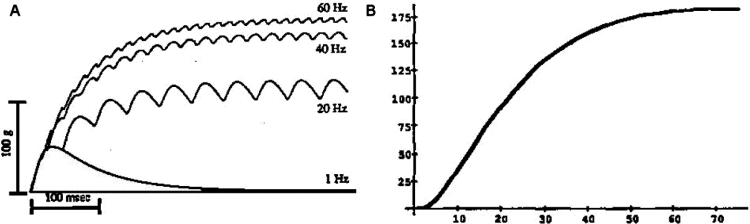Figure 2. AnimatLab Muscle Model. Adapted from (Shadmehr and Arbib 1992).

When motor neurons fire they release acetylcholine at the neuromuscular junctions that depolarizes the sarcomela. This wave of depolarization rushes through the T-tubule system to the sarcoplasmic reticulum where calcium ions are released and act on the myofibrils to elicit contractions (Becker, Kleinsmith et al. 2000). An impulse stimulation produces an isolated twitch shown as the single bump at 1 Hz in figure 3. As the motor neuron fires faster these twitches begin to merge together until finally they reach some maximum value and the muscle is said to have reached tetanus, or maximal activation. If one plots the maximum tension that can be developed at each stimulus frequency a sigmoidal curve such as the one shown in part b of figure 3 can be produced that relates the strength of the stimulus to the maximum tension. Typically there will be multiple motor neurons involved in stimulating a given muscle. The more of these neurons that are firing the more fibers in the muscle are recruited and the larger the force that is produced.

This behavior is not explicitly modeled in AnimatLab. This is one of the biggest difference between the (Shadmehr and Wise; Shadmehr and Arbib 1992) muscle implementation and the one in AnimatLab. They explicitly model calcium activation of the muscle. Instead, in AnimatLab a non-spiking neuron is used to emulate the membrane of the muscle. Motor neurons connect to the non-spiking neuron to excite or inhibit it. The time constant of that neuron then determines how those inputs are integrated. This is related to force using an adapter. The membrane voltage of the non-spiking neuron is converted into an active tension that is applied at A in figure 1. The equation that does this is a sigmoidal function like the one seen below. You can configure the stimulus-tension of the muscle by selecting the Stimulus-Tension property to display its gain dialog.Figure 3. Stimulus-Tension Dialog for configuring the stimulus-tension curve.

## Length-Tension Relationship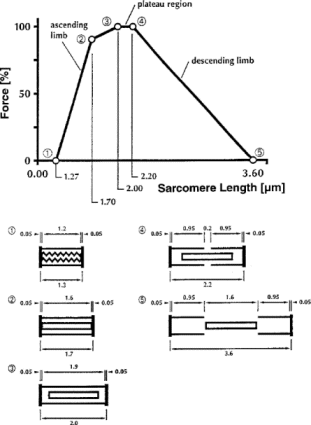Figure 4. Displays the relationship between the length of an individual sarcomere and the tension it develops. It also depicts the amount of overlap between the thick and thin elements of the sarcomere at different points along the curve. Adapted from (Rassier et al., 1999)

Within muscle there is also a relationship between the length of the muscle and the amount of active tension that it can develop (Ramsey and Street 1940.; Gordon, Huxley et al. 1966). This relationship is due to the fact that the level of tension is directly related to the number of crossbridge attachments that can be formed within each individual sarcomere in the muscle tissue. At the resting length there is a maximum overlap of the thin filament with the crossbridge-bearing zone of the thick filaments. As the muscle is moved away from this resting length the amount of overlap decreases and thus decreases the amount of tension that this sarcomere can develop (Gordon, Huxley et al. 1966). Figure 4 displays this property of muscle with a graph that was originally produced by Gordon, Huxley and Julian. They built a device that allowed them to record the tension and length for an individual sarcomere. The first part of figure 4 shows this relationship, and the second part of the figure provides a graphic depiction of the overlap of elements within the sarcomere. Maximum tension is developed in the plateau region between segments 3 and 4 where there is maximum overlap.

Within AnimatLab this relationship is determined by the Length-Tension property. When you select that property it opens the dialog shown below. The resting length specifies the natural resting length of the muscle. Lwidth determines how much the the generated force attenuates for increases or decreases in the muscle length. Increasing Lwidth spreads out the inverted quadratic function and means that length changes attenuate the force level less.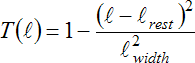Figure 5. Formula for calculating the amount of active tension that can be applied at the current length of the muscle.

The equations output value should be between 0 and 100%. This is the percentage of the stimulus force that is actually applied to the muscle. This means that the stimulus-tension curve we just talked about is modulated by this length-tension curve. If the muscle is stretched allot then regardless of the stimulus strength, the amount of force it can apply will be reduced depending on the length-tension relationship.Figure 6. Length-Tension Dialog.

## Velocity-Tension Relationship

A person is able to lift a light load much more quickly than they can a heavy load. You can quickly snatch a coin off the ground, but a 30 lb. rock will require more effort and take longer. Part of the explanation for this is the inertia of the load, but the primary factor in what causes this is that muscle is able to exert its maximum force when its velocity of shortening is zero. Since lifting the heavy item requires the production of a lot more force, the muscle must contract more slowly. The relationship between the velocity of shortening and the developed tension was first characterized by A. V. Hill in the 1930’s while working on frog muscles (Hill 1938). He fit the data to a rectangular hyperbola using equation 1. It has since become the standard model for this behavior of muscle and is used widely throughout the literature.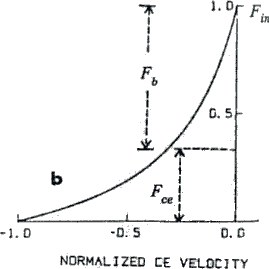Figure 7. Hill Force-Velocity Relationship. Adapted from (Winters, 1990)
 (F + a)(v + b) = (Fmax + a)b (1) af = a/Fmax = b/vmax (2)

The muscle model used in AnimatLab uses a simpler linear approximation instead of the true hyperbolic equation. This is why the name of this muscle is Linear Hill muscle. We specify a linear damping coefficient in parameter B. For most instances this approximation produces a behavior that is good enough for biomechanical modeling, especially if you are simulating numerous muscles that all work together. However, if you need a more detailed muscle model you should keep this approximation in mind. It may be that you will need to add a more detailed model to AnimatLab. We have plans to implement a more realistic model at some point, but it has not been a high priority for us. Luckily, others can add their own models and share those with other AnimatLab users.

## Mathematical Muscle Model

The differential equation used to simulate the muscle is shown below. To see how this is derived please see (Shadmehr and Wise; Shadmehr and Arbib 1992).Figure 8. Differential equation used to model muscle.

 T Muscle Tension. A Active tension applied by stimulation of membrane voltage. Kse Serial spring constant (SE). Kpe Parallel spring constant (PE). B Damping Coefficient. X Muscle Length.

## Using Muscles

You can add a muscle by selecting Linear Hill Muscle as the default body part type in the command toolbar. Then select the Add Bodies button and click on an existing part. This will add a new muscle to the biomechanical organism. However, it will not be visible yet. It will show up in the treeview though. For the muscle to become visible you must tell it which attachment points you want to string it between. A muscle can be connected to numerous attachment points. This allows you to simulate instances where the muscle may bend around other body parts.

A good example of this is shown in the locust leg in figure 1. The flexor muscle in the tibia does not connect directly from the femur to the tibia. Instead, the muscle is pulled over a lump and then connects to the tibia. This changes the angle of the application of force on the tibia and allows the much smaller flexor muscle to keep the leg flexed against the larger force generated in the extensor muscle. (The flexor muscle can only generate around 0.7 N of force, whereas the extensor muscle can generate around 15 N of force!!!). You can produce this same type of behavior in AnimatLab by using three attachment points and stringing the muscle between these points. Once this is done then tension is produced that goes from the tibia attachment towards the lump attachment instead of directly towards the femur attachment. Forces are also applied at each of the attachments that balance the forces at the ends. So if you have a 1 N contraction from the tibia connection to the lump then 1 N forces will also be applied at the lump attachment going towards the tibia attachment and the femur attachment.Figure 9. How forces are applied at attachment points.## Generating a Desired Tension

Determing the correct stimulus level that will generate a desired tension can be a difficult proposition. It requires using the inverse of the functions that the muscle uses to calculate tension. Here is a mathematical description of how to do this.

The first step is to figure out the Active tension that will be required to produce the tension you need. If you solve the tension equation for active tension you can find this value.Figure 10. Formula for calculating the amount of active tension that will be required to produce a desired tension.

Next, we need to take into consideration the effect of the length-tension curve. As the muscle is stretched away from its resting length less of the active force is applied, reducing the amount of tension that can be developed by the muscle. We need to multiply the active tension by the inverse of the length-tension percentage in order to increase it to a level that will overcome the attenuation due to stretching of the muscle.Figure 11. Formula for calculating the amount of active tension needed to produce a desired tension while taking into consideration the effects of the length-tension curve.

Finally, we need to work out the inverse of the stimulus-tension curve and use that to determined the correct stimulus voltage that will produce the desired active tension. The following table lists each variable in the equation.

 Variable Description A The active tension that needs to be generated. A1 X-offset of the stimulus-tension curve. A2 Amplitude of the stimulus-tension curve. A3 Steepness of the stimulus-tension curve. V Voltage stimulus needed to produce the desired tension.Figure 12. Formula for calculating the voltage stimulus needed to generate a desired tension.

Fortuanetly, there is a simpler way of doing this. The muscle has a property called Calc Stimulus that opens a dialog where these values can be calculated automatically using the current settings of the muscle. Please see the help info on this property for more details.

## Golgi Tendon Organs

Golgi tendon organs measure the tension in a muscle and provide this as a Type Ib sensory feedback signal. This information is used in feedback systems to maintain limb stiffness and esnure that the muscle does not attempt to produce to much force to ensure that the muscle is not damaged. The muscle has a Type Ib firing rate constant. This constant is multiplied with the tension of the muscle to determine the type Ib firing rate. This firing rate is a sensory signal that is provided by the muscle that you can use in a feedback loop.

## Muscle Properties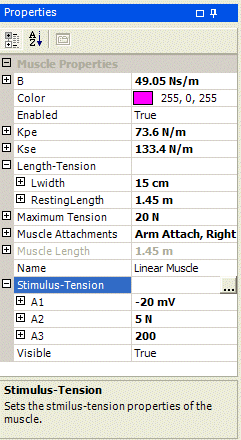Figure 13. The properties of the muscle.
B
The damping coefficient. This determines the how much resistive, or viscous, force is generated when the muscle length changes. This is a linear parameter. The force-velocity relationship in this muscle model is a linear approximation of the true hyper-bolic relationship seen in real muscle.
Default value: 1 Ns/m
Acceptable range: Any value greater than or equal to 0.

Calc Stimulus
This is a dialog that lets you calculate the muscle membrane voltage that will be required to produce a given tension using the current settings of the muscle. This is a rather complex calculation that is detailed here. You must calculate the amount of active tension that will need to be applied and use the inverse of the stimulus-tension curve to perform this calculation. This dialog was added to allow you to make these calculations automatically. When you click on this property a button with ellipses is shown. When you click that button it opens the dialog. There are places for you to enter the desired tension, the offset of the muscle from its natural length where you want this tension to develop, the velocity of shortening of the muscle, and the derivative of the tension. When you hit the calculate button it performs the calculations and fills in the the tension length percentage for this offset, activation tension, and the membrane voltage that will be required assuming a polynomial gain slope of 1. Keep in mind that there are a lot of assumptions in these calculations that will probably not hold for long in the dynamic simulation, so this should be used to give you a good idea of the tension that will be developed. As the muscle length changes or forces are applied the tension in the muscle will move away from this value. So use these values to help get yourself in the correct ballpark, and then refine them as needed.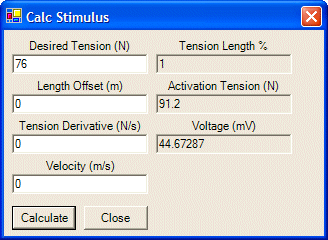Color
The color of the body.  The user can enter a color value using R (Red) G (Green) B (Blue) values or they can use the color chooser.   To use the color chooser, click on the color property and a dropdown arrow will appear..  Click the drop down arrow to open up the color chooser and then select the desired color.Default value:  Red (255, 0, 0)
Acceptable range:  R: 0 - 255, G: 0 - 255, B: 0 - 255;
Enabled
Enables or disables the muscle. If this is true then tensions generated are applied at the attachment positions. If it is false then no tensions are calculated and none are applied.

Default value: True
Acceptable range:  True/False

Ib Discharge Constant
This is a constant that is multiplied by the tension of the muscle to determine the spikes per second for the Ib fibers.
Default value: 100 Spikes/sN
Acceptable range: Any value greater than or equal to 0.

Kpe
The spring constant for the parallel spring.

Default value: 1 N/m
Acceptable range:  Any value greater than 0.

Kse
The spring constant for the serial spring.

Default value: 10 N/m
Acceptable range:  Any value greater than or equal to 0.

Lwidth
Determines how broad the length-tension curve is. The curve is an inverted quadratic centered at the natural length. The larger this value is the broader the curve, and the less attenuation of the applied force there is for a given change in length.

Default value: 50 cm
Acceptable range:  Any value greater than or equal to 0.

Natural Length
The natural length of the muscle. Changes from this length lead to decreases in the amount of applied tension according to the length-tension curve. This value also determines the delta X used in the differential equation.

Default value: 100 cm. When you set the attachments the first time this is set to the current length of the muscle.
Acceptable range:  Any value greater than 0.

Maximum Tension
The maximum tension that this muscle can apply.
Default value: 100 N
Acceptable range:
any number greater than zero

Muscle Attachments
Determines which muscle attachments this muscle is connected to.  When you select this property an ellipsis will appear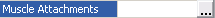.  When you click on an ellipsis the muscle attachment dialog will appear.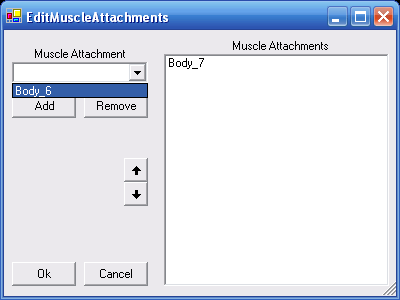This dialog allows you to specify which muscle attachments the muscle is connected to.  Using the drop down list you select available muscle attachments and press add.  The list box on the right tells you which muscle attachments are already added.  The up and down arrows allow you to change the order in which the muscle attachments are added.
Acceptable values:  Any muscle attachments on the biomechanical organism

Muscle Length
A read-only property that tells you the length of this muscle. This is the total length between all attachment positions.

Pe Length Percentage
This is the percentage of the muscle resting length that is the Pe section. The Se section is whatever is left over. This is important in determining discharge rates in stretch receptors.

Default value: 90 %
Acceptable range: Any value between 1 and 100.

Minimum Pe Length Percentage
This is the minimum length the Pe section can attain. This is to prevent the Pe section from going all the way to 0, and the Se section from growing longer than the muscle length. This determines how much total tension the muscle can generate and is important in determining discharge rates in stretch receptors.

Default value: 5 %
Acceptable range: Any value between 1 and Pe Length Percentage.

Stimulus-Tension A1
This is the horizontal offset of the center of the sigmoidal function used to convert membrane voltage into applied tension. Use this to center the curve.
Default value: -40 mv
Acceptable range: Any value.

Stimulus-Tension A2
The maximum applied tension for the stimulus-tension curve.
Default value: 5 N
Acceptable range: Any value greater than 0.

Stimulus-Tension A3
The steepness of the curve. If this value is larger then the curve goes from the minimum to maximum faster than if the value is smaller.
Default value: 100
Acceptable range: Any value greater than 0.

Visible
Determines if this muscle is visible in the simulation.
Default value: True
Acceptable range: True/False.